Next: Linearity Up: Blind Deconvolution of HST Previous: Introduction

# Application to Simulated Data

The algorithm has been successfully applied to both real and simulated astronomical data (Jefferies &Christou, 1993; Jefferies 1993) and its ability to accurately preserve photometry in the observed image has been demonstrated. In this section we quantify this photometric linearity by application to the simulated wide field camera star cluster data made available through ST ScI (Hanisch, 1993).

## Noiseless Case

In order to investigate the algorithm's performance without the influence of detector and photon noise we took the ``truth'' data set and convolved it with the given PSF to generate a noiseless image. We then reduced this using the Fourier modulus constraint and extracted the true object distribution and PSF. The restored object showed no residuals. This demonstrated the ability of the algorithm to extract both object and PSF and also the linearity of the restored object.

## Single Object Input

The algorithm was applied to the ``sim1'' data. In the blind-deconvolution mode the algorithm does not recover the true PSF with any fidelity. However, extra information is contained within the Fourier modulus which is obtained from the simple quotient of the Fourier components of the object and the given PSF. Thus we used the algorithm in its phase retrieval mode. The initial object and PSF estimates were the observed objects and PSF respectively. Fig. 1 shows the convolution image, ``sim1'', and Fig. 2 shows the reconstructed object and PSF using the Fourier modulus constraint. Note the reduction of the extended ``halos'' in the object sources which are now ``point-sources.''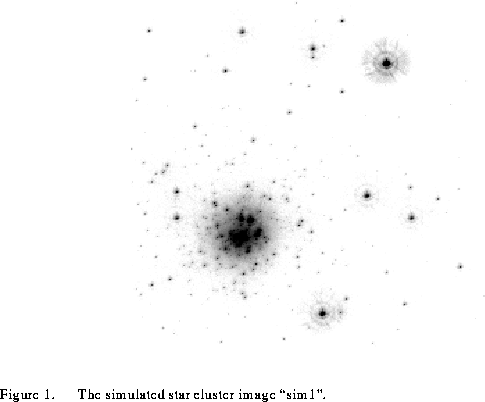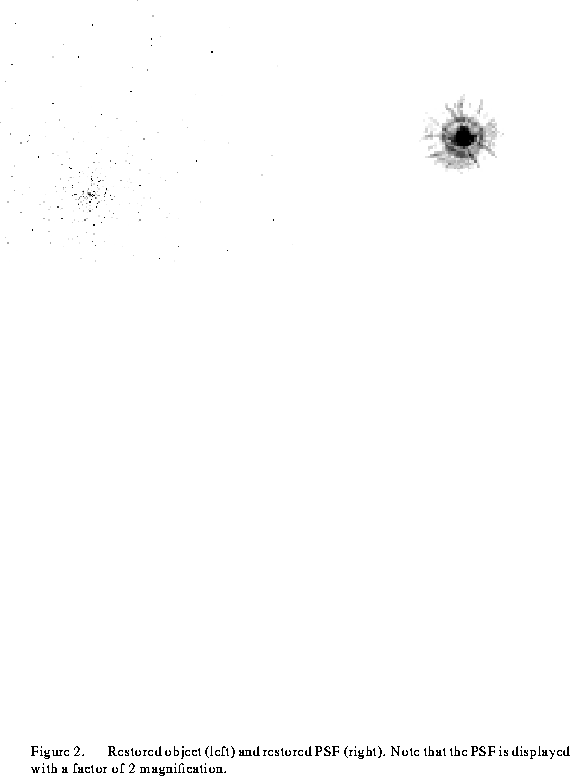## Two Object Input

For the next experiment we applied the algorithm in its blind deconvolution mode to the images ``sim1'' and ``sim2'' simultaneously. These two images have different noise realizations and a slightly different PSF. We used one of the convolution images as the initial object estimate and the brightest well isolated star, to the image top right, as the PSF estimates. The restored object and the two restored PSF's are shown in Fig. 3. Note that the PSFs show strong Airy rings as well as the filaments but have not converged to the measured PSF result. However, the object shows single pixel sources.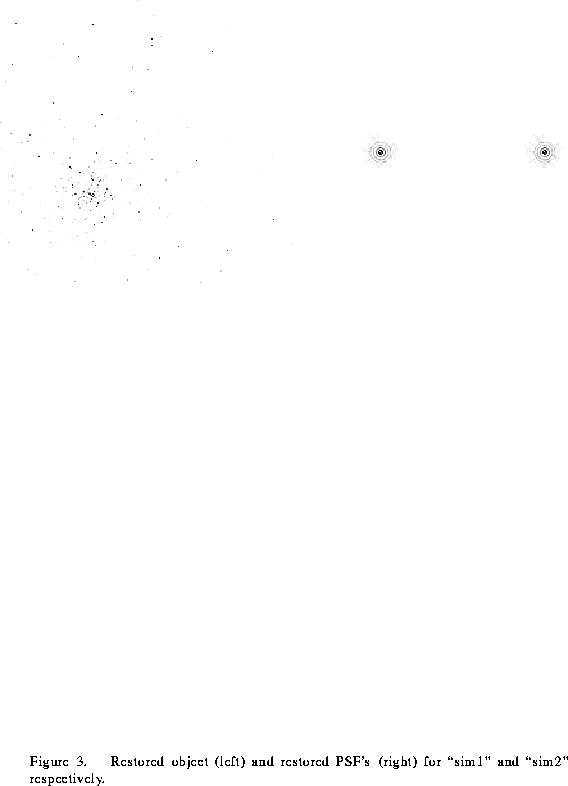## Four Object Input

For this experiment we increased the number of multiple images from two to four using the previous two images along with two images of the simulated star cluster obtained with WFPC 2, i.e. ``sim1c'' and ``sim2c''. We thus had four independent observations of the same field, each with a different PSF and noise realizations. As for the previous case, the algorithm was run as a blind deconvolution using one of the WFPC 2 images as the initial object estimate and the brightest star in each image as the corresponding PSF estimates. Due to the sizes of images, each of them 256
256, there were in excess of 300,000 variables to minimize. The nature of the problem forced us to reduce these data on the San Diego Supercomputer Center Cray YMP/2. The restored object and PSFs are shown in Fig. 4. Note the noise in the restored PSFs. The algorithm has found a solution which places the noise into the four different PSFs as it is not systematic and is therefore not common to the object. These PSFs are noisier than those restored for the two previous cases.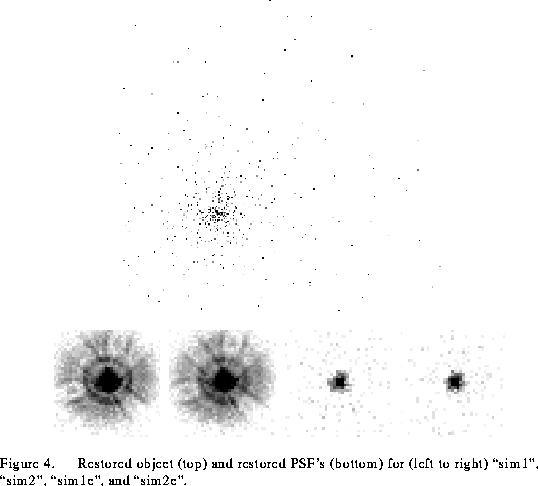Next: Linearity Up: Blind Deconvolution of HST Previous: Introduction

rlw@sundog.stsci.edu
Mon Apr 18 14:59:01 EDT 1994